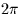Next: SLA_DRANGE - Put Angle into Range
Up: SUBPROGRAM SPECIFICATIONS
Previous: SLA_DR2AF - Radians to Deg,Min,Sec,Frac

## SLA_DR2TF - Radians to Hour,Min,Sec,Frac

ACTION:
Convert an angle in radians to hours, minutes, seconds, fraction (double precision).

CALL:
CALL sla_DR2TF (NDP, ANGLE, SIGN, IHMSF)

GIVEN:

 I NDP number of decimal places of seconds ANGLE D angle in radians

RETURNED:

 C SIGN +' or -' IHMSF I(4) hours, minutes, seconds, fraction

NOTES:
1.
NDP less than zero is interpreted as zero.
2.
The largest useful value for NDP is determined by the size of ANGLE, the format of DOUBLE PRECISION floating-point numbers on the target machine, and the risk of overflowing IHMSF(4). For example, on a VAX computer, for ANGLE up to, the available floating-point precision corresponds roughly to NDP=12. However, the practical limit is NDP=9, set by the capacity of the 32-bit integer IHMSF(4).
3.
The absolute value of ANGLE may exceed. In cases where it does not, it is up to the caller to test for and handle the case where ANGLE is very nearlyand rounds up to 24 hours, by testing for IHMSF(1)=24 and setting IHMSF(1-4) to zero.Next: SLA_DRANGE - Put Angle into Range
Up: SUBPROGRAM SPECIFICATIONS
Previous: SLA_DR2AF - Radians to Deg,Min,Sec,Frac

SLALIB --- Positional Astronomy Library